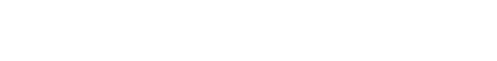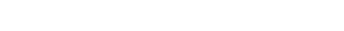# Number Sense for the GMAT

What is number sense and how can you recognize number sense problems on the GMAT? Before we get into the details, let’s start with a few number sense practice problems. Remember, no calculator.## Warm-Up Problems1) Rank those three in order from smallest to biggest.

(A) I, II, III
(B) I, III, II
(C) II, I, III
(D) II, III, I
(E) III, I, II

2) Let P = 36000.  Let Q equal the sum of all the factors of 36000, not including 36000 itself.  Let R be the sum of all the prime numbers less than 36000.  Rank the numbers P, Q, and R in numerical order from smallest to biggest.

(A) P, Q, R

(B) P, R, Q

(C) Q, P, R

(D) R, P, Q

(E) R, Q, P3) Rank those three in order from smallest to biggest.

(A) I, II, III
(B) I, III, II
(C) II, I, III
(D) II, III, I
(E) III, II, I

Solutions for these number sense problems will come at the end of this blog article.

## What is Number Sense?

Many GMAT Quantitative Problems, like the foregoing pair, test number sense.  What is number sense?  Number sense is a good intuition for what happens to different kinds of numbers (positive, negative, fractions, etc.) when you perform various arithmetic operations on them.

Number sense is what allows some folks to “see” shortcuts such as estimation or visual solutions.  For example, in any of the problems above, there’s absolutely no need to do any detailed calculations: in fact, folks with number sense can probably do all the math they need to do in their heads.

## Examples of a few number sense facts

1. Making the numerator of a fraction bigger makes the whole fraction bigger.
2. Making the denominator of a fraction bigger makes the whole fraction smaller.
3. (big positive) + (small negative) = something positive
4. (small positive) + (big negative) = something negative
5. Multiplying by a positive decimal less than one makes something smaller.
6. Dividing by a positive decimal less than one makes something bigger.

Of course, it would be near impossible to make anything like a complete list.  The left-brain reductionist dreams of something like an exhaustive list one could study, but number sense is all about right-brain pattern matching.   If you’re not familiar with the distinction of left/right hemisphere, see this GMAT post which touches on similar issues.

## How do you get number sense?

If you don’t have it, how do you get it?  That’s not an easy question.  There’s no magical shortcut to number sense, but here are some concrete suggestions.

1. Do only mental math.  You shouldn’t be using a calculator to practice for the GMAT anyway.  Try to do simpler math problems without even writing anything down.   Furthermore, look for opportunities every day, in every situation, to do some simple math or simple estimation (e.g. there are about 20 cartons of milk on the grocery store’s shelf—about how much would it cost to buy all twenty?)

2. Look for patterns with numbers.  Add & subtract & multiply & divide all kinds of numbers—positive integers, negative integers, positive fractions, negative fractions, and look for patterns. Number sense is all about pattern with numbers!! 🙂

3. This is a BIG one—in any GMAT practice problem that seemed (to you) to demand incredibly long calculations, but which had a very elegant solution of which you would have never dreamt—that problem & its solution are pure gold.  In a journal, write down what insights were used to simplify the problem dramatically.  Force yourself to articulate this, and return to this solution and to your notes on it often.  Over time, you should develop an array of problems like this, and if you study those solutions, you probably will start to see patterns.

4. Similar to #3: search the two forums,GMAT Club and Beat the GMAT, for similarly difficult questions, and look for elegant solutions.  That’s a great place to ask the experts (including yours truly) for more detailed explanations of their choices in the solution.

5. Here’s a variant on a game you can play, alone or with others who also want practice.  Pick four single digit numbers at random—some repeats are allowed.   You could roll a die four times, and use the results.  Now, once you have those four numbers, your job is to use all four of them, each of them only once, and any arithmetic, to generate each number from 1 to 20.  By “any arithmetic,” I mean any combination of: (a) add, subtract, multiply, divide; (b) exponents; and (c) parentheses & fractions

For example, if the four numbers I picked were {1, 2, 3, 4}, I could get 2 fromFor any one number, you only need to come up with it in one way (although you can consider it a bonus to come up with multiple ways for a single number!)  Here, I show three ways just to demonstrate the possibilities.  A few examples for some of the higher numbers:Notice that I used a variant of the expression for 13 to create an expression for 14.  Also, if I changed the plus sign in the expression for 13 to a minus sign, I would get an expression for 11.  Also, notice that if 1 is one of the four numbers, then if you don’t need it, you can simply multiply by it; furthermore, notice in the expression for 13 and in the second expression for 2 above, the exponent of 1 is a useful place to stash other numbers you don’t need!

As you practice, you will start to develop a sense of how expressions for one number can be tweaked to give you another number.   Overall, using similar combinations, you have to get every number from 1-20 with these four, or with whatever four you pick.   Actually, the set {1, 2, 3, 4} is a very good warm-up set.  When you want more of a challenge, use {2,3,3,5}.   🙂

One of our Remote Test Prep Experts, Jeff Derrenberger, created an awesome web game based on this mental math game. Click on the banner to check it out!## Practice Number Sense Problem

Here’s a practice number sense problem. If you didn’t get anywhere with the practice problem, you may want to study the solution below carefully.

## Practice Problem Solution

1) Notice that all three of these are close to fractions that equal 1/3.  The fractions that equal 1/3 would be, respectively, 50/150, 110/330, and 300/900.   First of all, only the second one has a higher numerator, so the second one is more than 1/3 and the other two are less than 1/3.  Therefore, II is the greatest.

Now, from I and III, which is greater?  Well, think about it this way.  50/150 = 300/900, because both of those equal 1/3.   How much less than one third is each one of these?  Well, 47/150 is 3/150 less than 50/150 = 1/3, and 299/900 is 1/900 less than 300/900 = 1/3.  Well, clearly, 3/150 > 1/900 (the latter has a smaller numerator and a larger denominator!)  Therefore, starting from 1/3, 47/150 goes down further than does 299/900.  Therefore, 47/150, dropping down a larger distance, must be the minimum value.  Therefore, the correct order is I, III, II.

2) We know that some of the factors of 36,000 are 18,000, 12,000, and 9,000.  Right there, those three add up to 39,000 more than 36,000.  Right there, we know that P < Q.  We can eliminate (C) and (E).

Now, R is a little trickier.  We don’t need to have detailed knowledge here.  We know there are several prime numbers less than 100.  Obviously, the density of prime numbers gets slightly less as we get bigger.  Let’s assume, extremely conservatively, that when we get up into the 20 and 30 thousands, there is at least one prime number every thousand: one between 20K and 21K, one between 21K and 22K, all the way up to 36K.  The 6 primes in the thirty thousands are all greater than 30K, so let’s estimate their sum as (30K)*6 = 180K.  The 10 primes in the twenty thousands are all greater than 20K, so let’s estimate their sum as (20K)*10 = 200K.  Right away, that’s 380K on an extremely conservative estimate–we didn’t even include any of the primes less than 20,000.  There is no way that the sum of the factors of 36K, not including 36K itself, is going to be more than ten times 36K!  Thus, R is much larger than Q, and the correct order is P < Q < R.

BTW, if your curious, according to Wolfram Alpha, the sum of the factors of 36000, not including 36000, is Q = 91,764, and the sum of all the prime number less than 36000 is R = 64,711,067.

3) Here, we have to “un-simplify” the square-roots to get a sense of their relative size.From this, we see that II is less than I.  From this alone, we can eliminate (A) & (B).

The trickier item on the list is III.  Without a calculator, it would be nearly impossible compute an exact value for the fourth-root of 401.  But consider this: the fourth root of a number is the number to the power of 1/4, and 1/4 = (1/2)*(1/2), so the fourth root is the square root of a square root.  Now, of course, 401 is not itself a perfect square, but it is very close to a perfect square.This demonstrates that III. is slightly larger than I.   Therefore, the order from least to greatest is II, I, III.

•